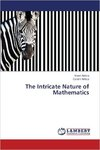## College of Arts & Sciences Faculty Books#### Title

The Intricate Nature of Mathematics

#### Description

This book emerged from a set of lecture notes used by one of the authors to teach a 200 level course in Nature of Mathematics. The course was introduced as a bridge from traditional Calculus I, II, III courses to higher level courses of Mathematics, such as Abstract Algebra and Advanced Calculus. The book introduces basic notions from set theory, symbolic logic, functions and relations, number theory, combinatorics and graph theory. It also gives an introduction to more abstract mathematical proofs. The techniques discussed in the book include: direct proof, in particular proof by enumeration of cases, proof by contradiction, proof by mathematical induction, proofs using Well Ordering Principle, pigeonhole principle, inclusion-exclusion principle and coloring arguments.

978-3659424434

7-11-2013

#### City

Saarbrücken, Germany

#### Disciplines

MathematicsCOinS